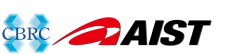MAFFT version 7

# Multiple alignment program for amino acid or nucleotide sequences

## Acceptable symbols in the default mode

• Protein sequences can contain:
• 20 standard amino acid characters (A, R, N, D, C, Q, E, G, H, I, L, K, M, F, P, S, T, W, Y, V)
• Unknown (X)
• Ambiguous amino acids (B, Z, J, X) and period (.); scored equivalently to X
• Both uppercase and lowercase are accepted and not distinguished.  Automatically converted to uppercase in the output.

• Nucleotide:
• a, c, g, t, u
• Ambiguous nucleotides (r, y, w, s, k, m, d, v, h, b; IUPAC-IUB codes) can be used and are scored as:
• score(r,a) = ( score(a,a) + score(g,a) ) / 2
score(r,g) = ( score(a,g) + score(g,g) ) / 2
score(r,t) = ( score(a,t) + score(g,t) ) / 2
score(r,c) = ( score(a,c) + score(g,c) ) / 2
...
score(r,y) = ( score(r,t) + score(r,c) ) / 2
...
score(r,r) = ( score(a,a) + score(g,g) ) / 2
score(y,y) = ( score(c,c) + score(t,t) ) / 2
...
• Both uppercase and lowercase are accepted and not distinguished.  Automatically converted to lowercase in the output.

If other alphabets are included in the input data, then the calculation stops with an error message.  Non-alphabetical characters (excl. period) are removed by default.  Use the --anysymbol option below, if you need to use these characters.

Sequence type (protein / nucleotide) is automatically recognized based on the frequency of a, t, g, c, and u, unless the --amino or --nuc flag is given.

## --anysymbol

To use unusual characters (e.g., U as selenocysteine in protein sequence; i as inosine in nucleotide sequence), use the --anysymbol option:
```% mafft --anysymbol input > output
```

It accepts any printable characters (U, O, #, \$, %, etc.; 0x21-0x7e in the ASCII code), execpt for > (0x3e) and ( (0x28).  Unusual characters are scored as unknown (not considered in the calculation), unlike in the --text mode

When the input data is:

```>
SampleSequenceWithUnusualCharacter
>
Sample#Sequence_With%Various^Unusual*Characters
>
SAMPLESEQUENCE
```

The result will be:

```>
Sample-Sequence-With---------Unusual-Character-
>
Sample#Sequence_With%Various^Unusual*Characters
>
SAMPLE-SEQUENCE--------------------------------
```

Upper/lower case is preserved.  The --anysymbol option is internally equivalent to the --preservecase option.

For aligning non-biological sequences, use the --text mode, in which unusual characters are also considered in the alignment calculation.